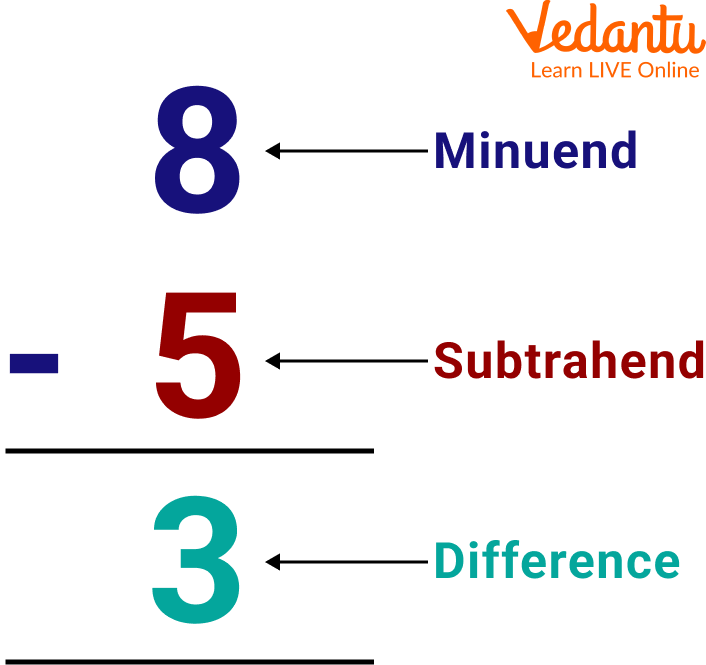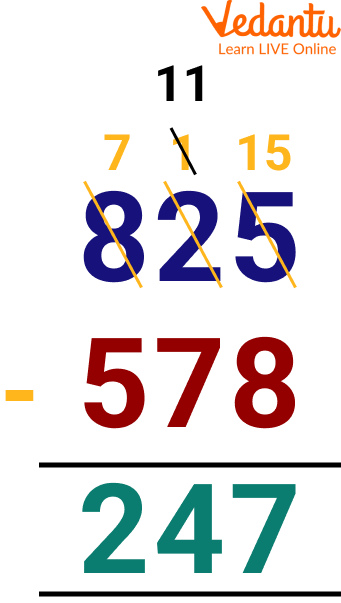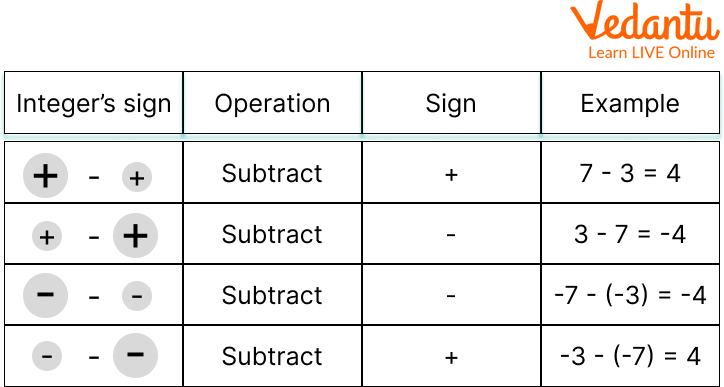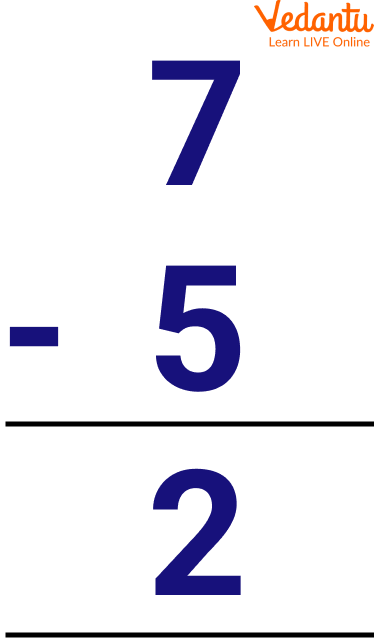Courses
Courses for Kids
Free study material
Free LIVE classes
More

# Subtraction for Kids## Introduction to Subtraction

Subtraction is one of the four basic arithmetical operations being used in mathematics for solving problems. The process of subtraction always starts from the right side of a number and moves to the left like addition. Subtraction is the process of taking away a number from another. It is a primary arithmetic operation that is denoted by a subtraction symbol (-) and is the method of calculating the difference between two numbers. This article will help kids in building their concepts about what subtraction is and how to subtract two numbers using the rules of subtraction. This uses images and examples to illustrate the topic deeply and differently. So, Let's begin with the understanding of our topic.

## Define Single Digit Subtraction

A single-digit subtraction involves only a one-digit number i.e. 0 to 9 to subtract a number from the other one-digit number. Some basic terms used in subtraction include Minuend, which is the larger number from which the smaller number, known as the subtrahend, is to be subtracted. The result of their subtraction is known as the difference.Process of Subtraction

## Rules of Subtraction

Various rules to be followed in performing subtraction are given below:

1. Check whether the given top number is smaller or larger than the bottom number.

2. If the given top number is greater than the bottom number, then subtraction starts from the right digit of the number and goes on till the last digit.Subtraction of a Greater Number with a Smaller Number

1. If the given top number is smaller than the bottom number then subtraction takes place by borrowing one from the next digit on the left.Subtraction of Greater Number with Smaller Number by Taking Carry

1. Further, repeat step 2 to subtract the entire quantities.

## Subtraction Sign Rules

Rules for taking the signs in case of subtraction are given below:

1. When both numbers are negative, the result will always be positive.

2. When any one of the numbers is positive and the other is negative, the result will depend on the sign of the greater number.

3. When both numbers are positive, the result will always be positive.Subtraction Sign Rules

## Subtraction Examples

Some examples of subtraction for kids are discussed below:

Example 1: Subtract 1 from 6

Ans: As 6 is a greater number, so subtract 1 from 6, and we get 5 as the difference.

Example 2: Subtract 5 from 7

Ans: As 7 is a greater number, so subtract 5 from 7, and write the answer.Showing Subtraction of Two Whole Numbers

Thus the difference between 7 and 5 is 2.

## Single Digit Subtraction Worksheets

Single-digit subtraction worksheets are given below:

1. 6 - 3 = (Ans: 3)

2. 3 - 2 = (Ans: 1)

3. 4 - 3 = (Ans: 1)

4. 5 - 1 = (Ans: 4)

## Practice Problems

Some of the practice problems are assigned to students to master the concept of subtraction.

Q 1. 8 - 2

Ans: 6

Q 2. 18 - 0

Ans: 18

Q 3. 7 - 7

Ans: 0

Q 4. 9 - 8

Ans: 1

Q 5. 11 - 3

Ans: 8

## Summary

Subtraction is one of the most basic and important operations used in maths. It creates a strong base for children in calculating the quantities accurately. It has wide applications in one's daily life. Subtraction is the base of learning several topics in mathematics, namely place values, word problems, mensuration, etc. It helps children in developing their mental ability and problem-solving skills. This article has covered all the details about what is subtraction, and the rules for subtraction and single-digit subtraction. If in doubt, ask in the comments.

Last updated date: 23rd Sep 2023
Total views: 94.5k
Views today: 1.94k

## FAQs on Subtraction for Kids

1. What are the properties that subtraction follows?

Subtraction follows four basic properties namely:

• Closure Property

• Identity Property

• Inverse Property

• Distributive Property of Multiplication over Subtraction

2. Is it possible to subtract two integers of the same signs?

Yes, it is quite possible to subtract two integers of the same signs. For example 4 - 2 = 1 and 5 - 2 = 3.

3. How do you subtract three-digit numbers?

The subtraction of three-digit numbers is the same as that of two digits numbers. It includes these steps:

• Write the numbers in columns form

• Check for the top number, if it is greater than the bottom number, then start subtracting from the right and move toward left

• If the top element is smaller than the bottom number then borrow one carry from the next digit and proceed with the subtraction

• Continue to subtract the digits till the last digit in the leftmost part is found